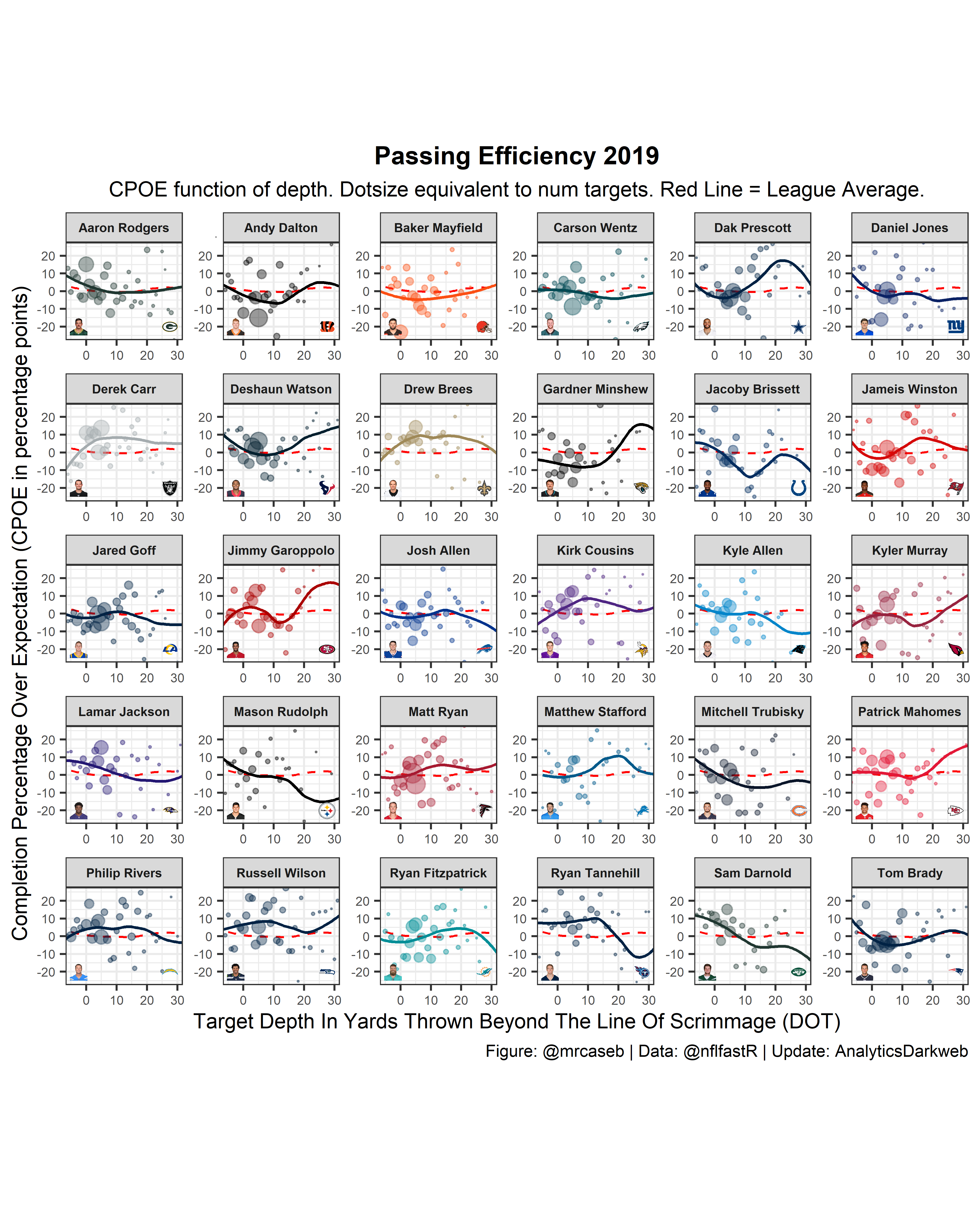# Matching players without ID keys

Rebuilding player graphs when ID keys go missing or are corrupted.

08-19-2020

Sometimes when we go to remake graphs from other people resources that used to work no longer do. This example shows how to work through that problem.

First let’s load the data and define which season we care about.

``````
library(tidyverse)
library(nflfastR)
seasons <- 2019
pbp <- purrr::map_df(seasons, function(x) {
glue::glue("https://raw.githubusercontent.com/guga31bb/nflfastR-data/master/data/play_by_play_{x}.csv.gz")
)
})

roster <-
filter(teamPlayers.position == "QB", team.season == 2019)

cpoe <-
pbp %>%
filter(!is.na(cpoe)) %>%
group_by(passer_player_id, air_yards) %>%
summarise(
count = n(),
cpoe = mean(cpoe)
)

season <- 2019``````

Next, we have a couple of players that are in our Top 30 from last year that have changed teams from where our roster has a different nfl ID structure than what we get from nflfastR.

So instead we can match on first initial, last name, and jersey number. I’ve renamed posteam to team.abbr below to change as little of Seb’s code as possible. Also, we have to update the roster file to indicate that the Raiders have moved to Las Vegas.

``````
summary <-
pbp %>%
separate(passer_player_name, c("firstName", "lastName"), sep = "\\.") %>%
filter(!is.na(cpoe)) %>%
group_by(lastName, posteam, firstName, passer_player_id, jersey_number) %>%
summarise(plays = n()) %>%
ungroup() %>%
arrange(desc(plays)) %>%
mutate(
lastName = ifelse(lastName == "Minshew II", "Minshew", lastName)
) %>%
left_join(
roster %>%
filter(team.season == seasons) %>%
mutate(firstInit = str_extract(teamPlayers.firstName, "\\w"), team.abbr = ifelse(team.abbr == "OAK", "LV", team.abbr)) %>%
select(name = teamPlayers.displayName, firstInit, teamPlayers.lastName, team.abbr, teamPlayers.headshot_url, teamPlayers.jerseyNumber),
by = c("lastName" = "teamPlayers.lastName", "firstName" = "firstInit", "jersey_number" = "teamPlayers.jerseyNumber", "posteam" = "team.abbr")
) %>%
mutate(# some headshot urls are broken. They are checked here and set to a default
"http://static.nfl.com/static/content/public/image/fantasy/transparent/200x200/default.png",
)
) %>%
left_join(cpoe, by = "passer_player_id") %>%
left_join(
teams_colors_logos %>% select(team_abbr, team_color, team_logo_espn),
by = c("posteam" = "team_abbr")
) %>%
rename(team.abbr = posteam)

colors_raw <-
summary %>%
group_by(passer_player_id) %>%
summarise(team = first(team.abbr), name = first(name)) %>%
left_join(
teams_colors_logos %>% select(team_abbr, team_color),
by = c("team" = "team_abbr")
) %>%
arrange(name)

n_eval <- 80
colors <-
as.data.frame(lapply(colors_raw, rep, n_eval)) %>%
arrange(name)

mean <-
summary %>%
group_by(air_yards) %>%
summarise(league = mean(cpoe), league_count = n())``````

Next, we need to change how Sebastian calls for colors in the geom_smooth call due to a package update. We can make a named vector to match the color hex numbers to the data frame.

``````
asp <- 1.2
cols <- c()
for(i in 1:length(unique(summary\$team_color))) {
cols <- append(cols, unique(summary\$team_color)[i])
}
color_names <- as.vector(unique(summary\$team_color))
cols <- set_names(cols, color_names)

plot <-
summary %>%
ggplot(aes(x = air_yards, y = cpoe)) +
geom_smooth(
data = mean, aes(x = air_yards, y = league, weight = league_count), n = n_eval,
color = "red", alpha = 0.7, se = FALSE, size = 0.5, linetype = "dashed"
) +
geom_smooth(
se = FALSE, alpha = 0.7, aes(weight = count, color = team_color), size = 0.65,
n = n_eval
) +
scale_color_manual(values = cols) +
geom_point(color = summary\$team_color, size = summary\$count / 15, alpha = 0.4) +
ggimage::geom_image(aes(x = 27.5, y = -20, image = team_logo_espn),
size = .15, by = "width", asp = asp
) +
ggimage::geom_image(aes(x = -2.5, y = -20, image = teamPlayers.headshot_url),
size = .15, by = "width", asp = asp
) +
xlim(-10, 40) + # makes sure the smoothing algorithm is evaluated between -10 and 40
coord_cartesian(xlim = c(-5, 30), ylim = c(-25, 25)) + # 'zoom in'
labs(
x = "Target Depth In Yards Thrown Beyond The Line Of Scrimmage (DOT)",
y = "Completion Percentage Over Expectation (CPOE in percentage points)",
caption = "Figure: @mrcaseb | Data: @nflfastR | Update: AnalyticsDarkweb",
title = glue::glue("Passing Efficiency {season}"),
subtitle = "CPOE function of depth. Dotsize equivalent to num targets. Red Line = League Average."
) +
theme_bw() +
theme(
axis.title = element_text(size = 10),
axis.text = element_text(size = 6),
plot.title = element_text(size = 12, hjust = 0.5, face = "bold"),
plot.subtitle = element_text(size = 10, hjust = 0.5),
plot.caption = element_text(size = 8),
legend.title = element_text(size = 8),
legend.text = element_text(size = 6),
strip.text = element_text(size = 6, hjust = 0.5, face = "bold"),
aspect.ratio = 1 / asp,
legend.position = "none"
) +
facet_wrap(vars(name), ncol = 6, scales = "free")``````

Lastly, we can kick out a save of our image file as before.

``````
plot````````````
ggsave(glue::glue("cpoe_vs_dot_{season}.png"), dpi = 600, width = 24, height = 21, units = "cm")``````

### Corrections

If you see mistakes or want to suggest changes, please create an issue on the source repository.

### Reuse

Text and figures are licensed under Creative Commons Attribution CC BY-NC 4.0. Source code is available at https://github.com/nflverse/open-source-football, unless otherwise noted. The figures that have been reused from other sources don't fall under this license and can be recognized by a note in their caption: "Figure from ...".

### Citation

`Darkweb (2020, Aug. 19). Open Source Football: Matching players without ID keys. Retrieved from https://www.opensourcefootball.com/posts/2020-08-19-matching-players-without-id-keys/`
```@misc{darkweb2020matching,